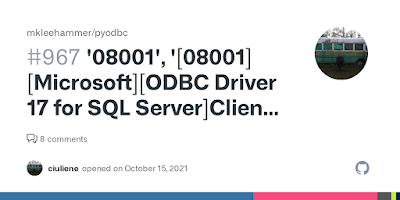## Saturday, 8 October 2011

PHP's interpretation of "overloading" is different than most object oriented languages. Overloading traditionally provides the ability to have multiple methods with the same name but different quantities and types of arguments. In PHP it's achieved through magic methods.

Remember :
1) All overloading methods must be defined as public.
2) None of the arguments of these magic methods can be passed by reference.

In PHP we have following two types of overloading and their respective functions.

Property Overloading : Property overloading is achieved through __get(), __set(), __isset() and __unset() funtions.

Property overloading only works in object context. These magic methods will not be triggered in static context. Therefore these methods should not be declared static.

`<?php require_once "DB.php"; class Persistable {        private \$data = array();     private \$table = "users";        public function __construct(\$user) {               \$this->dbh  = DB::Connect("mysql://user:password@localhost/database");        \$query      = "SELECT id, name, email, country FROM " .\$this->table . " WHERE name = ?";        \$this->data = \$this->dbh->getRow(\$query, array(\$user),DB_FETCHMODE_ASSOC);    }       public function __get(\$member) {               if (isset(\$this->data[\$member])) {            return \$this->data[\$member];        }    }       public function __set(\$member, \$value) {               // The ID of the dataset is read-only        if (\$member == "id") {            return;        }               if (isset(\$this->data[\$member])) {            \$this->data[\$member] = \$value;        }    }       public function __destruct() {               \$query = "UPDATE " . \$this->table . " SET name = ?,email = ?, country = ? WHERE id = ?";        \$this->dbh->query(\$query, \$this->name, \$this->email,\$this->country, \$this->id);    }}\$class = new Persistable("Martin Jansen");\$class->name = "John Doe";\$class->country = "United States";\$class->email = "john@example.com";?>`

The code defines two special methods called __get() and __set(). __get() is called when reading the value of an undefined property, and __set() is called when changing the same property's value.

This means that whenever someone reads or writes an undefined property from the persistable class, the special methods manipulate the information in the \$data property array instead of changing the class properties directly. (Remember: \$data contains the row from the database!)

For Example :

`<?php     require_once "DB.php";    class Persistable {                private \$data = array();         private \$table = "users";         public function __construct(\$user) {                    \$this->dbh = DB::Connect("mysql://user:password@localhost/database");            \$query = "SELECT id, name, email, country FROM " .\$this->table . " WHERE name = ?";            \$this->data = \$this->dbh->getRow(\$query, array(\$user),DB_FETCHMODE_ASSOC);        }        public function __get(\$member) {            if (isset(\$this->data[\$member])) {                return \$this->data[\$member];            }        }               public function __set(\$member, \$value) {                   // The ID of the dataset is read-only            if (\$member == "id") {                return;            }            if (isset(\$this->data[\$member])) {                \$this->data[\$member] = \$value;            }        }               public function __destruct() {            \$query = "UPDATE " . \$this->table . " SET name = ?, email = ?, country = ? WHERE id = ?";            \$this->dbh->query(\$query, \$this->name, \$this->email, \$this->country, \$this->id);        }    }       \$class = new Persistable("Martin Jansen");    \$class->name = "John Doe";    \$class->country = "United States";    \$class->email = "john@example.com";?>`
``` ```